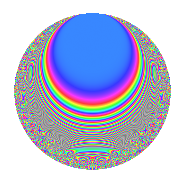# Properties

 Label 2005.2.a.fLevel 2005 Weight 2 Character orbit 2005.a Self dual Yes Analytic conductor 16.010 Analytic rank 0 Dimension 37 CM No

# Related objects

## Newspace parameters

 Level: $$N$$ = $$2005 = 5 \cdot 401$$ Weight: $$k$$ = $$2$$ Character orbit: $$[\chi]$$ = 2005.a (trivial)

## Newform invariants

 Self dual: Yes Analytic conductor: $$16.0100056053$$ Analytic rank: $$0$$ Dimension: $$37$$ Fricke sign: $$-1$$ Sato-Tate group: $\mathrm{SU}(2)$

## $q$-expansion

The dimension is sufficiently large that we do not compute an algebraic $$q$$-expansion, but we have computed the trace expansion.

 $$\operatorname{Tr}(f)(q) =$$ $$37q + 7q^{2} + 3q^{3} + 43q^{4} - 37q^{5} + 8q^{6} - 16q^{7} + 21q^{8} + 54q^{9} + O(q^{10})$$ $$\operatorname{Tr}(f)(q) =$$ $$37q + 7q^{2} + 3q^{3} + 43q^{4} - 37q^{5} + 8q^{6} - 16q^{7} + 21q^{8} + 54q^{9} - 7q^{10} + 42q^{11} - 13q^{13} + 14q^{14} - 3q^{15} + 63q^{16} + 18q^{17} + 22q^{18} + 22q^{19} - 43q^{20} + 16q^{21} - 10q^{22} + 23q^{23} + 23q^{24} + 37q^{25} + 21q^{26} + 3q^{27} - 18q^{28} + 33q^{29} - 8q^{30} + 11q^{31} + 54q^{32} + 2q^{33} + 8q^{34} + 16q^{35} + 91q^{36} - 11q^{37} + 29q^{38} + 25q^{39} - 21q^{40} + 24q^{41} + 4q^{42} + 25q^{43} + 84q^{44} - 54q^{45} + 31q^{46} + 7q^{47} + 4q^{48} + 45q^{49} + 7q^{50} + 94q^{51} - 43q^{52} + 49q^{53} + 38q^{54} - 42q^{55} + 46q^{56} + 6q^{57} + 15q^{58} + 69q^{59} + 9q^{61} + 17q^{62} - 38q^{63} + 107q^{64} + 13q^{65} + 74q^{66} + 13q^{67} + 86q^{68} - 14q^{70} + 51q^{71} + 81q^{72} - 47q^{73} + 79q^{74} + 3q^{75} + 59q^{76} + 2q^{77} + 20q^{78} + 67q^{79} - 63q^{80} + 125q^{81} - 24q^{82} + 80q^{83} + 50q^{84} - 18q^{85} + 69q^{86} - 32q^{87} - 12q^{88} + 34q^{89} - 22q^{90} + 39q^{91} + 85q^{92} + q^{93} + 12q^{94} - 22q^{95} + 77q^{96} - 14q^{97} + 40q^{98} + 133q^{99} + O(q^{100})$$

## Embeddings

For each embedding $$\iota_m$$ of the coefficient field, the values $$\iota_m(a_n)$$ are shown below.

For more information on an embedded modular form you can click on its label.

Label $$a_{2}$$ $$a_{3}$$ $$a_{4}$$ $$a_{5}$$ $$a_{6}$$ $$a_{7}$$ $$a_{8}$$ $$a_{9}$$ $$a_{10}$$
1.1 −2.69709 −2.81518 5.27428 −1.00000 7.59279 −1.69501 −8.83103 4.92525 2.69709
1.2 −2.59156 0.254340 4.71619 −1.00000 −0.659137 −3.82594 −7.03917 −2.93531 2.59156
1.3 −2.45894 0.456001 4.04640 −1.00000 −1.12128 2.81754 −5.03197 −2.79206 2.45894
1.4 −2.34377 3.11940 3.49326 −1.00000 −7.31116 −3.06432 −3.49986 6.73066 2.34377
1.5 −2.29904 −2.37846 3.28561 −1.00000 5.46819 −1.14540 −2.95567 2.65708 2.29904
1.6 −2.05755 2.25661 2.23350 −1.00000 −4.64308 1.74407 −0.480444 2.09227 2.05755
1.7 −1.89780 0.675950 1.60165 −1.00000 −1.28282 −2.83198 0.755984 −2.54309 1.89780
1.8 −1.67554 −1.95480 0.807444 −1.00000 3.27536 1.89440 1.99818 0.821260 1.67554
1.9 −1.33194 −3.14709 −0.225946 −1.00000 4.19172 −4.67738 2.96482 6.90415 1.33194
1.10 −1.31166 2.95262 −0.279548 −1.00000 −3.87283 1.12020 2.98999 5.71797 1.31166
1.11 −1.26616 −0.778484 −0.396848 −1.00000 0.985683 −1.29163 3.03478 −2.39396 1.26616
1.12 −1.00292 −0.400121 −0.994154 −1.00000 0.401289 0.439579 3.00289 −2.83990 1.00292
1.13 −0.973849 1.32303 −1.05162 −1.00000 −1.28843 3.84920 2.97182 −1.24959 0.973849
1.14 −0.667454 −3.21238 −1.55450 −1.00000 2.14411 2.82900 2.37247 7.31937 0.667454
1.15 −0.481240 0.287327 −1.76841 −1.00000 −0.138273 −4.64922 1.81351 −2.91744 0.481240
1.16 −0.272348 1.38053 −1.92583 −1.00000 −0.375985 −0.00887442 1.06919 −1.09413 0.272348
1.17 −0.141163 1.61581 −1.98007 −1.00000 −0.228092 −0.325783 0.561837 −0.389145 0.141163
1.18 0.0750780 3.37448 −1.99436 −1.00000 0.253350 1.31500 −0.299889 8.38713 −0.0750780
1.19 0.210270 −2.86952 −1.95579 −1.00000 −0.603374 0.843239 −0.831782 5.23416 −0.210270
1.20 0.287516 0.183703 −1.91733 −1.00000 0.0528174 −5.18007 −1.12630 −2.96625 −0.287516
See all 37 embeddings
 $$n$$: e.g. 2-40 or 990-1000 Embeddings: e.g. 1-3 or 1.37 Significant digits: Format: Complex embeddings Normalized embeddings Satake parameters Satake angles

## Inner twists

This newform does not have CM; other inner twists have not been computed.

## Atkin-Lehner signs

$$p$$ Sign
$$5$$ $$1$$
$$401$$ $$-1$$

## Hecke kernels

This newform can be constructed as the intersection of the kernels of the following linear operators acting on $$S_{2}^{\mathrm{new}}(\Gamma_0(2005))$$:

 $$T_{2}^{37} - \cdots$$ $$T_{11}^{37} - \cdots$$